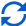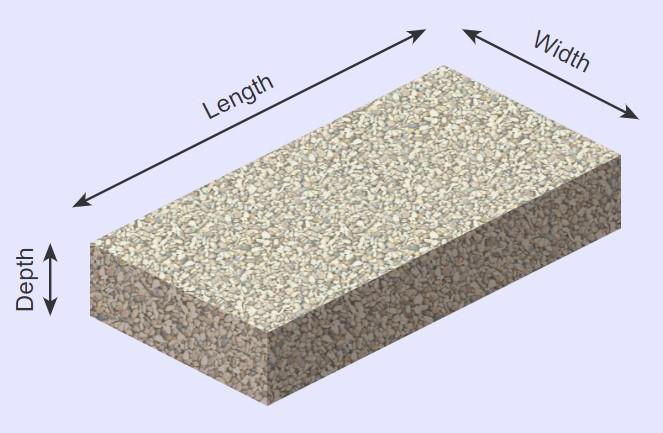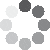Enter parameters LENGTH, WIDTH & DEPTH
Enter parameters
LENGTH, WIDTH & DEPTH
Length:
ft
• inches (in)
• feet (ft)
• yards (yd)
• centimeters (cm)
• meters (m)
Width:
ft
• inches (in)
• feet (ft)
• yards (yd)
• centimeters (cm)
• meters (m)
Depth:
in
• inches (in)
• feet (ft)
• yards (yd)
• centimeters (cm)
• meters (m)
Density:
105 - Gravel lb/ft³
• Gravel - 105 lb/ft³
• Concrete - 150 lb/ft³
• Limestone - 160 lb/ft³
• Sandstone - 145 lb/ft³
• Granite - 168 lb/ft³
• Marble - 160 lb/ft³
• Slate - 168 lb/ft³
• Basalt - 188 lb/ft³
• Crushed stone - 100 lb/ft³
• Sand - 100 lb/ft³
• Topsoil - 110 lb/ft³
• Custom
Price per unit of mass:
t
• pound (lb)
• US short ton (t)
• imperial-long ton (long t)
• kilogram (kg)
Price per unit of volume:
yd³
• cubic yards (yd³)
• cubic feet (ft³)
• cubic inches (in³)
• cubic centimeters (cm³)
• cubic meters ()Result
Area:
0
ft²
• square feet (ft²)
• square inches (in²)
• square yards (yd²)
• square miles (mi²)
• acres (ac)
• square centimeters (cm²)
• square meters ()
Volume:
0
yd³
• cubic yards (yd³)
• cubic feet (ft³)
• cubic inches (in³)
• cubic centimeters (cm³)
• cubic meters ()
Weight:
0
t
• pounds (lb)
• US short tons(t)
• imperial-long tons (long t)
• kilograms (kg)
Cost:
$Enter parameters AREA & DEPTH Area: ft² • square feet (ft²) • square inches (in²) • square yards (yd²) • square miles (mi²) • acres (ac) • square centimeters (cm²) • square meters () Depth: in • inches (in) • feet (ft) • yards (yd) • centimeters (cm) • meters (m) Density: 105 - Gravel lb/ft³ • Gravel - 105 lb/ft³ • Concrete - 150 lb/ft³ • Limestone - 160 lb/ft³ • Sandstone - 145 lb/ft³ • Granite - 168 lb/ft³ • Marble - 160 lb/ft³ • Slate - 168 lb/ft³ • Basalt - 188 lb/ft³ • Crushed stone - 100 lb/ft³ • Sand - 100 lb/ft³ • Topsoil - 110 lb/ft³ • Custom Price per unit of mass: t • pounds (lb) • US short tons (t) • imperial-long tons (long t) • kilograms (kg) Price per unit of volume: yd³ • cubic yards (yd³) • cubic feet (ft³) • cubic inches (in³) • cubic centimeters (cm³) • cubic meters ()Result Volume: 0 yd³ • cubic yards (yd³) • cubic feet (ft³) • cubic inches (in³) • cubic centimeters (cm³) • cubic meters () Weight: 0 t • pounds (lb) • US short tons (t) • imperial-long tons (long t) • kilograms (kg) Cost:$
Enter parameters
VOLUME
Volume:
yd³
• cubic yards (yd³)
• cubic feet (ft³)
• cubic inches (in³)
• cubic centimeters (cm³)
• cubic meters ()
Density:
105 - Gravel lb/ft³
• Gravel - 105 lb/ft³
• Concrete - 150 lb/ft³
• Limestone - 160 lb/ft³
• Sandstone - 145 lb/ft³
• Granite - 168 lb/ft³
• Marble - 160 lb/ft³
• Slate - 168 lb/ft³
• Basalt - 188 lb/ft³
• Crushed stone - 100 lb/ft³
• Sand - 100 lb/ft³
• Topsoil - 110 lb/ft³
• Custom
Price per unit of mass:
t
• pounds (lb)
• US short tons (t)
• imperial-long tons (long t)
• kilograms (kg)
Price per unit of volume:
yd³
• cubic yards (yd³)
• cubic feet (ft³)
• cubic inches (in³)
• cubic centimeters (cm³)
• cubic meters ()Result
Weight:
0
t
• pounds (lb)
• US short tons (t)
• imperial-long tons (long t)
• kilograms (kg)
Cost:
$(No Ratings Yet)Loading... Similar Calculators: Real-time graphics. Make the calculations and see the changes. Real-time graphics. Make the calculations and see the changes. Real-time graphics. Make the calculations and see the changes. Embed Similar Calculators:Before you start any landscaping or home improvement project, you’ll agree that having an accurate estimate of the amount of material required is vital. You want this estimation to be as accurate as possible to ensure you don’t over or under order the material. That’s why we built our online material calculator. 😎 In this article, we will show you just how easy it is to use. In addition, we will walk you through a few full examples with the mathematical formulae the calculator uses. Our material calculator will make you more efficient and can even save you time and money! 👍 Contents: ## Material calculator formulaOur calculator first works out the base area and volume of the material required from the measurements you provide: $$Area = Length \times Width$$ $$Volume = Area \times Depth$$ In addition, if you know the density of the material, then you can calculate the weight of the required material: $$Weight = Density \times Volume$$ What if you don’t know the density? Well, this can easily be calculated using the formula: $$Density = {Mass \over Volume}$$ Want to know the best part? If you have the price per unit mass or price per unit volume for the material, then the material calculator can work out the total cost of the required material: $$Cost = Price\,Per\,Unit\,Mass \times Weight$$ or $$Cost = Price\,Per\,Unit\,Volume \times Volume$$ If you don’t know this information, then you can easily calculate the price per unit mass/volume for the material purchased using the formula: $$Price\,Per\,Unit\,Mass= {Total\,Cost\,of\,Material \over Weight\,of\,Material}$$ or $$Price\,Per\,Unit\,Volume = {Total\,Cost\,of\,Material \over Volume\,of\,Material}$$ Something not clear? 🤔 See below for three full examples where we calculate the volume, weight and total cost of materials starting with different initial criteria. Otherwise, simply enter your measurements and values into the calculator and get started on your landscaping project efficiently! ## Example calculations • Fill a space with standard 150 lb/ft³ concrete For our first example, let’s say we want to fill a space measuring 5 feet in length, 10 feet in width and 5 inches deep with standard concrete, which costs$50 per US short ton.

I want to calculate the total volume, weight and cost of the concrete required for this project.

I would enter these measurements into the online material calculator, which would perform the following operations:

$$Area = Length \times Width = 5\,ft \times 10\,ft = 50\,ft^2$$

$$Volume = Area \times Depth = 50\,ft^2 \times 5\,in = 20.833\,ft^3$$

$$Weight = Density \times Volume = 105\,lb/ft^3 \times 20.833\,ft^3 = 1.563\,t$$

$$Cost = Price\,Per\,Unit\,Mass \times Weight = 50\,/t \times 1.563\,t = 78.13$$

• Cover 12 square feet with custom aggregate

Imagine I want to cover 12 square feet of space with a custom material, ensuring equal coverage to a depth of 4 inches.

I am unsure of the material’s density, but it weighs 400 pounds for two cubic feet and costs $45 per US short ton. First, in order to calculate the density of the material I would do the following calculation: $$Density = {Mass \over Volume} = {400\,lb \over 2\,ft^3} = 200\,lb/ft^3$$ I would then enter all these measurements into the online calculator, selecting the appropriate units in order to calculate the volume, weight and cost: $$Volume = Area \times Depth = 12\,ft^2 \times 4\,in = 4\,ft^3$$ $$Weight = Density \times Volume = 200\,lb/ft^3 \times 4\,ft^3 = 0.4\,t$$ $$Cost = Price\,Per\,Unit\,Mass \times Weight = 45\,/t \times 0.4\,t = 18$$ • Fill 8 cubic feet with sand calculating price per unit volume For our final example, let’s say I want to fill a space measuring 8 cubic feet with sand (100 lb/ft³). I don’t know the price per unit volume of the sand, but I can purchase 10 cubic yards for$150.

I can therefore calculate the price per unit volume using the formula:

$$Price\,Per\,Unit\,Volume = {Total\,Cost\,of\,Sand \over Volume\,of\,Sand} = {150 \over 10\,yd^3} = 15\,/yd^3$$

I therefore enter all these parameters into the online material calculator to work out the total weight and cost of the required sand:

$$Weight = Density \times Volume = 100\,lb/ft^3 \times 8\,ft^3 = 800\,lb$$

$$Cost = Price\,Per\,Unit\,Volume \times Volume = 15\,/yd^3 \times 8\,ft^3 = 4.44$$

Finally, you might be wondering:

## What if I want to use different units?

Our online calculator can do the conversions for you, making it really easy to use different units for each measurement.

If you look back through our examples, you’ll notice that we do this.

For example, volume is given in cubic feet and price is dollars per cubic yard.

Simply select the appropriate unit in the drop down area and the calculator will do the conversion for you:

$$1\,foot = 12\,inches = 0.33\,yards = 30.48\,centimeters = 0.3048\,meters$$

$$1\,US\,short\,ton = 2000\,pounds = 0.893\,imperial\,long\,ton = 907\,kg$$

Embed this calculator on your site!Add live graphics
Copied to clipboard! Preview